# Kandidatexjobb i numerisk analys

## Projektförslag från numerisk analys

Kandidatsexjobbskursen på numerisk analys ska ge dig fördjupade kunskaper om utvalda numeriska beräkningsmetoder. Du kommer att få arbeta med ett större projekt med lärarstöd från forskare på avdelningen.

Numeriska beräkningsmetoder används inom många olika områden för att simulera olika fysikaliska processer. Det kan vara tex vågutbredning (akustiska, elektromagentiska, elastiska), strömningsmekanik (vätskor, gaser, plasma), analys av data, materialfysik, kemi, biologi, etc. I grunden handlar det om att med hjälp av datorn kunna lösa matematiska problem, ofta differentialekvationer, som formuleras inom dessa områden. Projektuppgiften i kandidatexjobbet kan knyta nära an till ett aktuellt forskningsprojekt inom gruppen men kan också vara en mer fristående problemstälning. Projekten inbegriper teoretisk analys, implementering och simuleringar av något tillämpat problem.

Exempel på genomförda projekt från tidigare år:

• Optimal Control of Inverted Pendulum, Oskar Stattin, 2015.
• Numerical Methods for Identification of Handwritten Digits: A singular value decomposition and transport problem approach, Helen From, Viktor Kull, 2017
• Construction of an Optimal Beam Using Newton's Method, Jiongrui Hu, Rebecca Wilson, 2015.
• Suspensions with small, spherical particles, Samuel Zackrisson, 2015.

Om du är intresserad av ett specifikt projekt nedan, kontakta handledaren och fråga om det finns platser kvar på det projektet.

## Quadrature based methods for Sylvester equations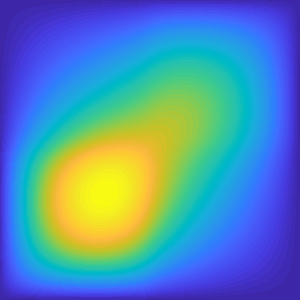This project concerns constructing new numerical methods for a problem called the Sylvester equation. The Sylvester equation arises in a variety of different contexts, such as dynamical systems and partial differential equations. In basic courses you have learned how to compute integrals numerically. Based on the integral formulations of the Sylvester of equations and numerical integration, the aim of this project is to develop new methods for computing (approximative) solutions to Sylvester equations

Handledare: Emil Ringh

## Numerical methods for subsurface flow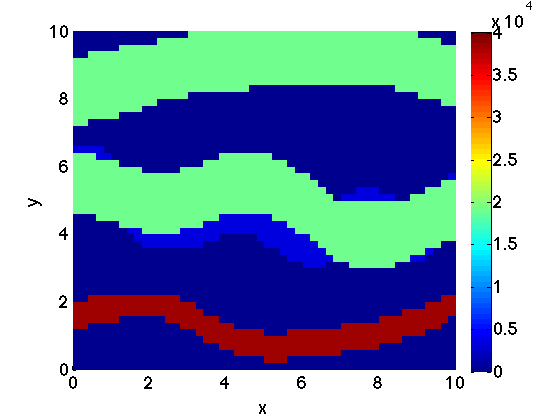Subsurface flow and transport are physical processes that describe how groundwater flows beneath the earth surface. These processes are also important in order to understand how geothermal energy can be extracted via water running through (and heated by) the hot subsurface rock. Different sedemntary layers may be more or less difficult for the water to flow through. This property, the permeability of the rock, can vary by orders of magnitudes making the construction of numerical methods for such problems challenging. In addition, standard numerical methods do not preserve the physics properly. In this project we consider how to construct numerical methods in such a way that the physics is preserved.

Handledare: Anna Nissen

## WoodpeckerMechanical systems of rigid bodies are often modelled by differential-algebraic systems. The woodpecker is a curious toy which features contact discontinuities. In practice one can observe that this toy leads to periodic oscillations. With this project, we try to model this behavior. Your task will be to develop a mathematical model and the corresponding numerical methods for simulating the woodpecker. In particular, you will show that stable oscillations exist and find the oscillation frequency.

Handledare: Michael Hanke

## Solving PDEs in “free space” by the use of FFTsIn many applications, one wants to numerically solve a partial differential equation (PDE) without imposing boundary conditions on an enclosing boundary and rather mimic an “infinite domain”. This is the case for example when simulating electromagnetic or acoustic waves that hit an object and scatters outwards. However, discretizing the PDE with traditional grid based techniques, a finite size domain must be used, and quite advanced techniques have been developed to avoid the artificial reflection that occurs at boundaries of the computational domain.

Handledare: Fredrik Fryklund och Anna-Karin Tornberg

## Approximation of the mean curvature vector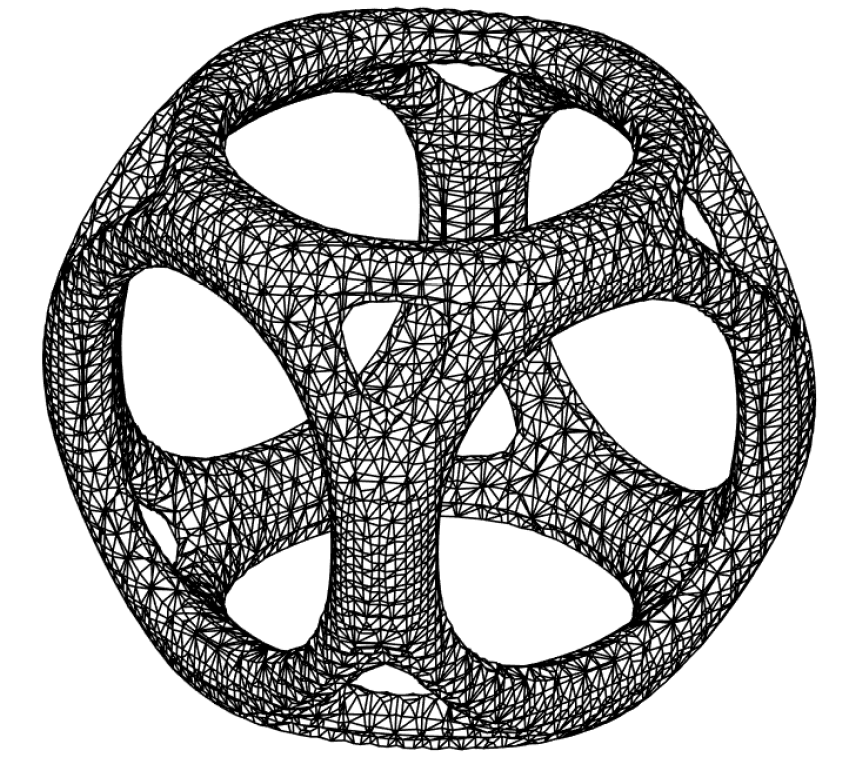Accurate computation of the mean curvature vector on a discrete surface plays an important role in computer graphics as well as in ce rtain surface evolution problems. An accurate approximation of the mean curvature vector is for example essential for the approximation of the surface tension force in simulations of multiphase flow problems. In this work we study a stabilized finite element method for approximation of the mean curvature vector.

Handledare: Sara Zahedi

## Can computers hear? - The mathematics of musicHave you ever wondered how a computer program can recognize music, voices or sounds in general? As we know sound waves are composed of several frequencies. To study the frequency content of sound waves, the Fourier transform can be used.

In this project you will write a computer program that can recognize individual musical notes generated by an instrument. Once the frequencies are recognized there are various possible applications and extensions.

Handledare: Joar Bagge and Aku Kammonen

## Multiscale methods for highly oscillatory ordinary differential equations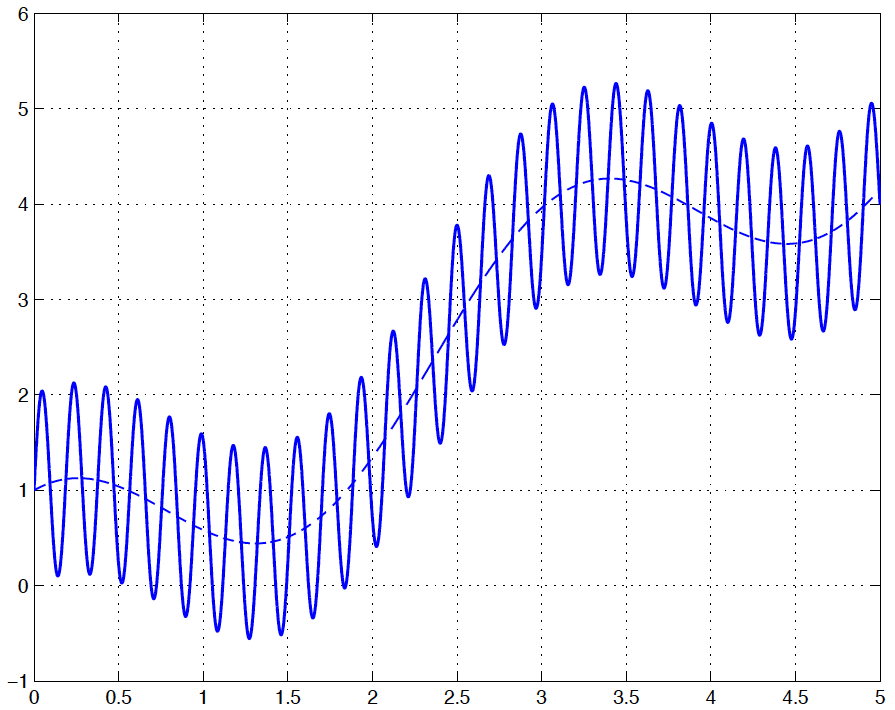In this project we consider ODEs with multiple time scales, i.e. ODEs whose solution consists of fast oscillations with period ε<<1, superimposed on a slowly varying function. The ODE problem is an example of a multiscale problem. They are common in many areas, from physics and chemistry to biology. They are characterized by the need to take very fine scale information into account even when only the coarse scales of the solution is of interest. This typically makes them very difficult to treat with numerical methods.

Handledare: Olof Runborg

## Numerical quadrature for singular integralsIntegrals with singular integrands occur for example when solving integral equations. In this project we will consider the numerical evaluation of integrals where the integrand is singular at some point within the integration interval, but where the integral is well defined. This requires special numerical quadrature schemes, and we will investigate some strategies to design such schemes. We will use a standard quadrature rule to evaluate a singular 1D integral and also analyze why the accuracy of the numerical integration degenerates for this case, and what accuracy can be expected.

Handledare: Anna-Karin Tornberg och Fredrik Fryklund

## Wave propagation in a heterogenous medium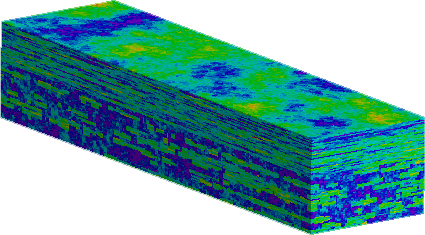Elastic wave equations have a significant relevance for determining subsurface formations in the earth’s crust. For instance, in order to recover oil or petroleum from geologic formations, to sequestrate carbon dioxide (i.e. storing liquid CO2 in the subsurface) or to predict earthquakes, scientists need a clear image of the geologic underground, i.e. its composition concerning rock formations, soil, faults, groundwater, oil, petroleum and so on. In this project you will learn how to approach these problems numerically.

Handledare: Patrick Henning

## Modern numerical algorithms for the pagerankModern web search engines such as Google, Yahoo and Bing Search sort the results of your query by measuring the importance of webpages containing your criteria. The algorithm used is called Pagerank and it was the first algorithm used by Google. The idea behind the Pagerank follows a simple logic: the importance of a page is roughly estimated by counting the number and quality of links that point to that page. In this project you will learn about this algorithm and how it can be improved to incorporate new information.

Handledare: Giampaolo Mele (och Elias Jarlebring)

## Trafik och folkträngsel med varierande framkomlighetAvsikten med detta projekt är att modellera och numeriskt lösa flöde av gång, cykel eller biltrafik, genom att numerisk lösa vissa partiella differentialekvationer.

Handledare: Anders Szepessy

## How accurate can you estimate the derivative from sampled data?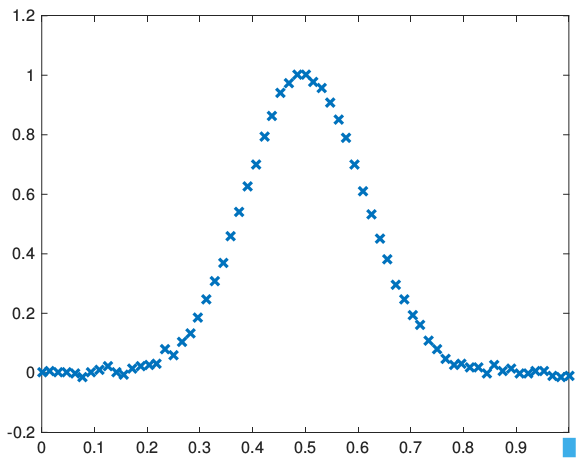In real world applications one is often confined to measured values. These measurements are subject to errors that are inherent in the physical process observed and the method to measure data. Therefore, the errors cannot be made as small as we wish. Since numerical differentiation is very sensitive with respect to errors in the data, special methods must be used in order to obtain meaningful approximations.

Handledare: Michael Hanke

## Efficient methods for matrix multiplicationIn your linear algebra course you have learned how you can compute the product of two matrices A and B with O(n3) operations, by computing scalar products of rows in A with the columns of B. In many computational simulations of accurate mathematical models, large parts of the computational effort is spent on such matrix-matrix products. In this project you will learn about other ways (such as with the strassen algorithm) to compute the matrix-matrix products, with less operations.

Handledare: Elias Jarlebring

## Dimensionering av vågkraftverksnätEtt sätt att utvinna energi är att utnyttja vågkraft. Det kan göras med WEC-bojar (Wave Energy Converters). Dagens bojar är dock dyra att bygga och ger förhållandevis lite energi per boj. Men om man kan förutsäga hur vågorna kommer att se ut den närmaste tiden, finns det sätt att konstruera bojen så att energi-utvinningen per boj kan ökas avsevärt. Tyvärr är vågor svåra att förutse och modellera. Ett sätt är då att lägga ut flera WEC-bojar i närheten av varandra så att den yttersta bojen kan förvarna de inre bojarna när och hur vågorna kommer. I detta projekt får du lära dig om numeriska metoder för att simulera detta.

Handledare: Ninni Carlsund Levin

## The discovery of NeptuneBeskrivning kommer senare

Handledare: Olof Runborg

Kolla på projekten igen på tunnelbanan hem: